# Right circular cone

The volume of a right circular cone is 5 liters. Calculate the volume of the two parts into which the cone is divided by a plane parallel to the base, one-third of the way down from the vertex to the base.

Correct result:

V1 =  0.1852 l
V2 =  4.8148 l

#### Solution: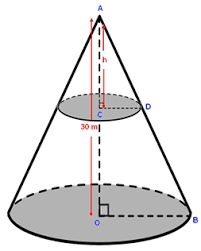We would be pleased if you find an error in the word problem, spelling mistakes, or inaccuracies and send it to us. Thank you!

Showing 1 comment:Dr Math
1:3 is the ratio of heights
1:32 = 1:9 is the ratio of the area of base circles... due to two dimensional nature of the area.
1:33 = 1:27 is the ratio of volumes...  .. volume has three-dimensional natureTips to related online calculators
Check out our ratio calculator.
Tip: Our volume units converter will help you with the conversion of volume units.

#### You need to know the following knowledge to solve this word math problem:

We encourage you to watch this tutorial video on this math problem:

## Next similar math problems:

• Volume per timeHow long does fill take for a pump with a volume flow of 200 l per minute fill a cube-shaped tank up to 75% of its height if the length of the cube edge is 4 m?
• The woodenThe wooden block measures 12 cm, 24 cm, and 30 cm. Peter wants to cut it into several identical cubes. At least how many cubes can he get?
• Into boxHow many cubes with an edge of 2.5 cm fit into a box measuring 11.6 cm; 8.9 cm and 13.75 cm?
• Cargo spaceThe cargo space of the truck has the dimensions a = 4.2 m, b = 1.9 m, c = 8.1 dm. Calculate its volume.
• Jansen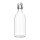Jansen had 1.3 litres of fruit juice. He drank 0.5 litres during lunch. How many litres of fruit juice does he have left?
• Sphere cutA sphere segment is cut off from a sphere k with radius r = 1. The volume of the sphere inscribed in this segment is equal to 1/6 of the volume of the segment. What is the distance of the cutting plane from the center of the sphere?
• AquariumHow many liters of water can fit in an aquarium measuring 30, 15 and 20 cm?
• Ice floe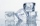What is the volume of an ice floe weighing 326 kg? The density of ice is only 920 kg/m3.
• The bodyThe body has dimensions of 2m 2dm and 10 cm. It weighs 28 kg. What is its density?
• Brick wallWhat is the weight of a solid brick wall that is 30 cm wide, 4 m long and 2 m high? The density of the brick is 1500 kg per cubic meter.
• Thousand ballsWe have to create a thousand balls from a sphere with a diameter of 1 m. What will be their radius?
• Gas sphere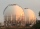The gas tank has the shape of a sphere with a diameter of 14 m. How many m3 of gas will fit in it?
• Clay balls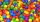How many clay balls with a radius of 1 cm can be made from a ball of clay with a radius of 8 cm?
• Equilateral coneWe pour so much water into a container that has the shape of an equilateral cone, the base of which has a radius r = 6 cm, that one-third of the volume of the cone is filled. How high will the water reach if we turn the cone upside down?Calculate the volume of a quadrilateral pyramid, the base of which has the shape of a rectangle with dimensions a = 6cm, b = 4cm and height v = 11cmThe cylinder has a surface area of 300 square meters, while the height of the cylinder is 12 m. Calculate the volume of this cylinder.How high does the water in the aquarium reach, if there are 36 liters of water in it? The length of the aquarium is 60 cm and the width is 4 dm.